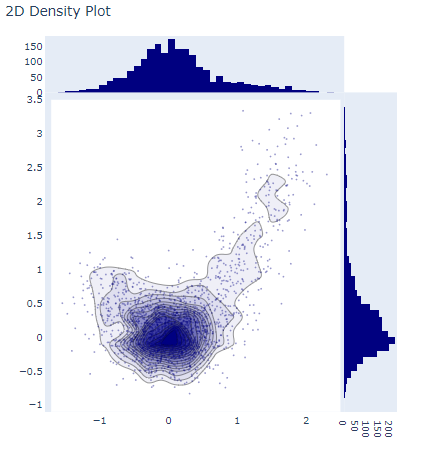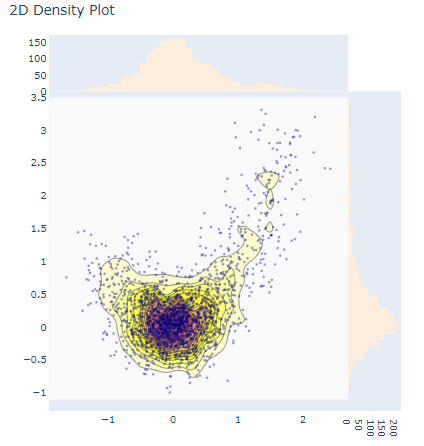# plotly.figure_factory.create_2d_density() function in Python

Plotly library of Python can be very useful for data visualization and understanding the data simply and easily.

## Plotly.figure_factory.create_2d_density

This function is used to create 2d density.

Syntax: plotly.figure_factory.create_2d_density(x, y, colorscale=’Earth’, ncontours=20, hist_color=(0, 0, 0.5), point_color=(0, 0, 0.5), point_size=2, title=’2D Density Plot’, height=600, width=600)

Parameters:

x: x-axis data for plot generation

y: y-axis data for plot generation

colorscale: An rgb or hex color, a color tuple or a list or tuple of colors.

hist_color: the color of the plotted histograms

point_color: the color of the scatter points

point_size: the color of the scatter points

title: set the title for the plot

height: the height of the chart

width: the width of the chart

Example 1:

## Python3

 `from` `plotly.figure_factory ``import` `create_2d_density ` `import` `numpy as np ` ` `  ` `  `t ``=` `np.linspace(``-``1``,``1.2``,``2000``) ` `x ``=` `(t``*``*``3``)``+``(``0.3``*``np.random.randn(``2000``)) ` `y ``=` `(t``*``*``6``)``+``(``0.3``*``np.random.randn(``2000``)) ` ` `  `fig ``=` `create_2d_density(x, y) ` `fig.show()`

Output:2D density plot

Example 2:

## Python3

 `from` `plotly.figure_factory ``import` `create_2d_density ` `import` `numpy as np ` ` `  ` `  `# Make data points ` `t ``=` `np.linspace(``-``1``,``1.2``,``2000``) ` `x ``=` `(t``*``*``3``)``+``(``0.3``*``np.random.randn(``2000``)) ` `y ``=` `(t``*``*``6``)``+``(``0.3``*``np.random.randn(``2000``)) ` ` `  `# Create custom colorscale ` `colorscale ``=` `[``'#7A4579'``, ``'#D56073'``, ``'rgb(236,158,105)'``, ` `             ``(``1``, ``1``, ``0.2``), (``0.98``,``0.98``,``0.98``)] ` ` `  `# Create a figure ` `fig ``=` `create_2d_density(x, y, colorscale``=``colorscale, ` `      ``hist_color``=``'rgb(255, 237, 222)'``, point_size``=``3``) ` ` `  `# Plot the data ` `fig.show()`

Output:2D Density plot using parameters

Whether you're preparing for your first job interview or aiming to upskill in this ever-evolving tech landscape, GeeksforGeeks Courses are your key to success. We provide top-quality content at affordable prices, all geared towards accelerating your growth in a time-bound manner. Join the millions we've already empowered, and we're here to do the same for you. Don't miss out - check it out now!

Previous
Next